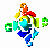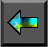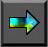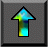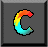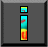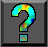Next: 6.12 Summary Up: 6 Fortran M Previous: 6.10 Performance Issues

# 6.11 Case Study: Fock Matrix Construction

Programs 6.13 and 6.14 sketch an FM   implementation of the parallel Fock matrix construction algorithm of Section 2.8. Recall that in this algorithm, P computation tasks must be able to read and write two large distributed data structures. This capability is achieved by encapsulating these data structures in separate data tasks. Each computation task repeatedly generates requests for data values and then performs computation. The FM program implements computation and data tasks as compute and data processes, respectively, and connects these processes using a many-to-many communication structure, as illustrated in Figure 6.10. This structure uses P mergers to link P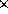P outports ( To) with P inports ( Ti). Each process is given an array of P outports, one connected to each merger. In Programs 6.13 and 6.14, P=128, and 1024 data values are allocated to P data tasks in a blocked fashion.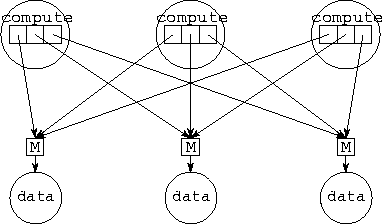Figure 6.10: FM implementation of the Fock matrix problem, with P=3. Each of the P compute processes has an array of P outports connected via mergers with P data processes.

For brevity, the FM programs presented here implement only an accumulate operation. A compute process wanting to accumulate a value to address addr sends a message containing an offset (the integer mod(addr,ND)) and the value to be accumulated (a real) on outport number addr/ND. Notice that the compute and data processes are similar in structure to the manager and worker of Program 6.5.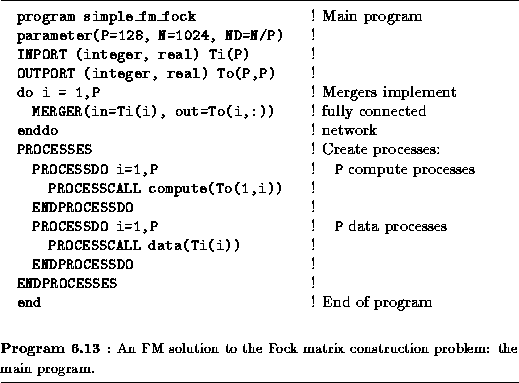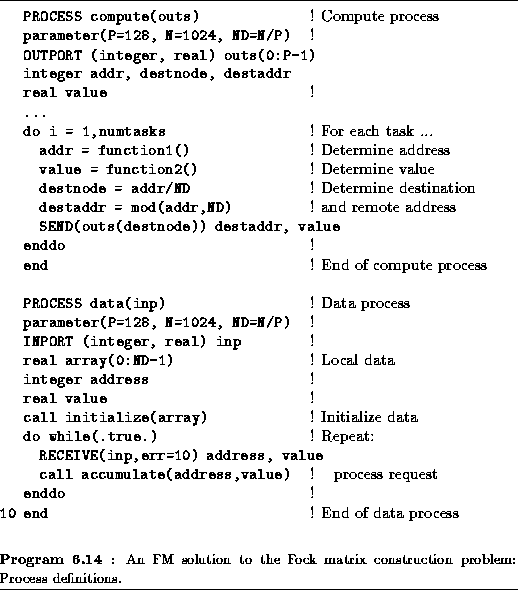Next: 6.12 Summary Up: 6 Fortran M Previous: 6.10 Performance Issues